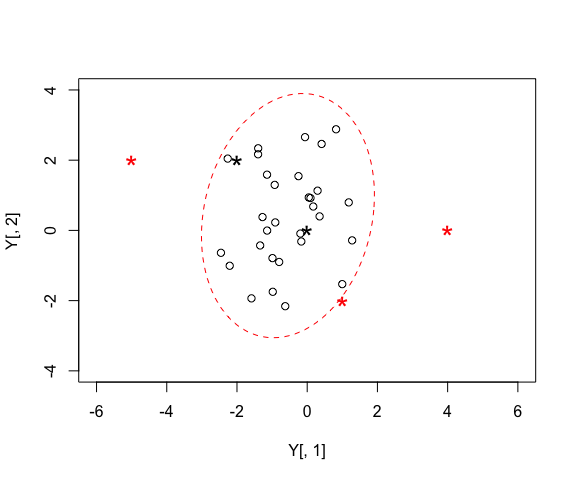# Points Inside or Outside Ellipse

## Creating some test data

First we will create some random data and draw an ellipse around it, and then we can test whether a defined set of other points are inside or outside the ellipse.


library(SIBER)

# set the random seed generator so we get consistent results each time
# we run this code.
set.seed(2)

# n random numbers
n <- 30

# some random multivariate data
Y <- generateSiberGroup(n.obs = 30)

##Determine whether points are inside or outside ellipse Now we want to determined whether a set of data points are inside or outside our ellipse. This could be the same data as used to generate the ellipse, and given that its a 95% prediction ellipse, we would expect there to be 95% of the data inside the ellipse on average. As it happens, with this random seed, all our data points are inside the 95% ellipse: stochasticity is so random. It could though be a set of other independent data points. The way the code works below is a bit of space-warping trickery. Essentially, there is a transformation that can be applied to our ellipse that makes it a perfect circle, centred around the origin (point [0,0]). We apply this transformation to both the ellipse boundary and also our data points that we want to test. It is then very easy to determine whether or not our points are within the radius of our circle or whether they are outside! Moving the ellipse to the origin is easy, as it just means subtracting the mean of the data. Warping the ellipse so it maps onto a circle is done by a bit of linear matrix algebra using the covariance matrix of the data (the same that defines the ellipse). SIBER has functions to help do this.


# plot this example data with column 2 by column 1
plot(Y[,2] ~ Y[,1], type = "p", asp = 1,
xlim = c(-4, 4),
ylim = c(-4, 4))

# add an ellipse, in this case a 95% ellipse
mu <- colMeans(Y) # centre of the ellipse
Sigma <- cov(Y) # covariance matrix of the ellipse

# percentile of the ellipse
p <- 0.95

# draw the ellipse
tmp <- addEllipse(mu, Sigma, p.interval = p, col = "red", lty = 2)

# Define a matrix of 5 data points to test against our ellipse.
# For ease of interpretation of this code, I have built this matrix by
# specifying each row on a separate line and do this by adding the option
# byrow = FALSE (by default R fills down the rows first of a matrix).
test.these <- matrix(c(-2,  2,
0,  0,
-5,  2,
1, -2,
4,  0),
byrow = TRUE,
ncol = 2, nrow = 5)

# transform these points onto ellipsoid coordinates
Z <- pointsToEllipsoid(test.these, Sigma, mu)

# determine whther or not these points are inside or outside the ellipse drawn
# with same p as above (percentile).
inside <- ellipseInOut(Z, p = p)

# inside points are marked TRUE which corresponds to 1 in numeric terms, and
# outside marked FALSE which corresponds to 0. So, below i set up my custom
# colour order of ("red", "black") and then look up [inside + 1] which will
# be [0 + 1 = 1 = "red"   for inside is FALSE] and
#    [1 + 1 = 2 = "black" for inside is TRUE].

# and plot them with colour coding for whether they are inside or outside
points(test.these[,2] ~ test.these[,1],
col = c("red","black")[inside + 1],
pch = "*",
cex = 2)##3 and more dimensions These functions will work just the same with more than 2 dimensions of data. In three dimensions you are testing whether your data are inside or outside a ball that has a transformation to and from an ellipsoid (spherical, cigar or frisbee shaped). The concept is exactly the same in 4 and more dimensions. The problem is illustrating the ellipsoid is not possible in >3 dimensions, and I have not yet implemented 3d versions of the ellipsoids, but I am sure many tutorials exist on how to plot these. Alternatively, and for illustrative purposes only you could plot each pair of your dimensions in turn, and add ellipses manually. The problem is that some points might appear to be in or out of the ellipse in these marginal plots, but might conflict with the true situation when all dimensions are considered simultaneously.

# set the random seed generator so we get consistent results each time
# we run this code.
#set.seed(2)

# n random numbers
n <- 10^4

# number of dimensions
d <- 3

# vector of d means between -1 and +1
mu <- stats::runif(d, -1, +1)

# a (d x d) covariance matrix
# pull a precision matrix from the wishart distribution and invert it to
# get the corresponding covariance matrix.
sigma <- solve(matrix(stats::rWishart(1, d, diag(d)), d, d))

# n-dimensional multivariate random numbers for this test
Y <- mnormt::rmnorm(n, mu, sigma)

# sample mean and covariance matrix
mu <- colMeans(Y) # centre of the ellipse
Sigma <- cov(Y) # covariance matrix of the ellipse

# percentile of the ellipsoid to test
p <- 0.95

# here i am just going to test whether my actual data points are inside
# or outside the 95% ellipsoid but you could replace with your own
# data points as above

# transform these points onto ellipsoid coordinates
Z <- pointsToEllipsoid(Y, Sigma, mu)

# determine whther or not these points are inside or outside the ellipse drawn
# with same p as above (percentile).
inside <- ellipseInOut(Z, p = p)

# how many of our points are inside our ellipse?
sum(inside) / length(inside)
#>  0.949